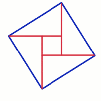#### You may also like### Fitting In

The largest square which fits into a circle is ABCD and EFGH is a square with G and H on the line CD and E and F on the circumference of the circle. Show that AB = 5EF. Similarly the largest equilateral triangle which fits into a circle is LMN and PQR is an equilateral triangle with P and Q on the line LM and R on the circumference of the circle. Show that LM = 3PQ### Pinned Squares

What is the total number of squares that can be made on a 5 by 5 geoboard?### Zig Zag

Four identical right angled triangles are drawn on the sides of a square. Two face out, two face in. Why do the four vertices marked with dots lie on one line?

# Just Opposite

##### Age 14 to 16Challenge Level$A$ and $C$ are the opposite vertices of a square $ABCD$, and have coordinates $(a,b)$ and $(c,d)$, respectively. What are the coordinates of the other two vertices? What is the area of the square? How generalisable are these results?